## 5.50. between_min_max

Origin

Used for defining $\mathrm{𝚌𝚞𝚖𝚞𝚕𝚊𝚝𝚒𝚟𝚎}_\mathrm{𝚌𝚘𝚗𝚟𝚎𝚡}$.

Constraint

$\mathrm{𝚋𝚎𝚝𝚠𝚎𝚎𝚗}_\mathrm{𝚖𝚒𝚗}_\mathrm{𝚖𝚊𝚡}\left(\mathrm{𝚅𝙰𝚁},\mathrm{𝚅𝙰𝚁𝙸𝙰𝙱𝙻𝙴𝚂}\right)$

Arguments
 $\mathrm{𝚅𝙰𝚁}$ $\mathrm{𝚍𝚟𝚊𝚛}$ $\mathrm{𝚅𝙰𝚁𝙸𝙰𝙱𝙻𝙴𝚂}$ $\mathrm{𝚌𝚘𝚕𝚕𝚎𝚌𝚝𝚒𝚘𝚗}\left(\mathrm{𝚟𝚊𝚛}-\mathrm{𝚍𝚟𝚊𝚛}\right)$
Restrictions
 $\mathrm{𝚛𝚎𝚚𝚞𝚒𝚛𝚎𝚍}$$\left(\mathrm{𝚅𝙰𝚁𝙸𝙰𝙱𝙻𝙴𝚂},\mathrm{𝚟𝚊𝚛}\right)$ $|\mathrm{𝚅𝙰𝚁𝙸𝙰𝙱𝙻𝙴𝚂}|>0$
Purpose

$\mathrm{𝚅𝙰𝚁}$ is greater than or equal to at least one variable of the collection $\mathrm{𝚅𝙰𝚁𝙸𝙰𝙱𝙻𝙴𝚂}$ and less than or equal to at least one variable of the collection $\mathrm{𝚅𝙰𝚁𝙸𝙰𝙱𝙻𝙴𝚂}$.

Example
 $\left(3,〈1,1,4,8〉\right)$ $\left(1,〈1,1,4,8〉\right)$ $\left(8,〈1,1,4,8〉\right)$

The first $\mathrm{𝚋𝚎𝚝𝚠𝚎𝚎𝚗}_\mathrm{𝚖𝚒𝚗}_\mathrm{𝚖𝚊𝚡}$ constraint holds since its first argument 3 is greater than or equal to the minimum value of the values of the collection $〈1,1,4,8〉$ and less than or equal to the maximum value of $〈1,1,4,8〉$.

Typical
 $|\mathrm{𝚅𝙰𝚁𝙸𝙰𝙱𝙻𝙴𝚂}|>1$ $\mathrm{𝚛𝚊𝚗𝚐𝚎}$$\left(\mathrm{𝚅𝙰𝚁𝙸𝙰𝙱𝙻𝙴𝚂}.\mathrm{𝚟𝚊𝚛}\right)>1$
Symmetries
• Items of $\mathrm{𝚅𝙰𝚁𝙸𝙰𝙱𝙻𝙴𝚂}$ are permutable.

• $\mathrm{𝚅𝙰𝚁}$ can be set to any value of $\mathrm{𝚅𝙰𝚁𝙸𝙰𝙱𝙻𝙴𝚂}.\mathrm{𝚟𝚊𝚛}$.

Arg. properties

Extensible wrt. $\mathrm{𝚅𝙰𝚁𝙸𝙰𝙱𝙻𝙴𝚂}$.

Reformulation

By introducing two extra variables $\mathrm{𝙼𝙸𝙽}$ and $\mathrm{𝙼𝙰𝚇}$, the $\mathrm{𝚋𝚎𝚝𝚠𝚎𝚎𝚗}_\mathrm{𝚖𝚒𝚗}_\mathrm{𝚖𝚊𝚡}$$\left(\mathrm{𝚅𝙰𝚁},\mathrm{𝚅𝙰𝚁𝙸𝙰𝙱𝙻𝙴𝚂}\right)$ constraint can be expressed in term of the following conjunction of constraints:

$\mathrm{𝚖𝚒𝚗𝚒𝚖𝚞𝚖}$$\left(\mathrm{𝙼𝙸𝙽},\mathrm{𝚅𝙰𝚁𝙸𝙰𝙱𝙻𝙴𝚂}\right)$,

$\mathrm{𝚖𝚊𝚡𝚒𝚖𝚞𝚖}$$\left(\mathrm{𝙼𝙰𝚇},\mathrm{𝚅𝙰𝚁𝙸𝙰𝙱𝙻𝙴𝚂}\right)$,

$\mathrm{𝚅𝙰𝚁}\ge \mathrm{𝙼𝙸𝙽}$,

$\mathrm{𝚅𝙰𝚁}\le \mathrm{𝙼𝙰𝚇}$.

Counting
 Length ($n$) 2 3 4 5 6 7 8 Solutions 17 184 2417 37806 689201 14376608 338051265

Number of solutions for $\mathrm{𝚋𝚎𝚝𝚠𝚎𝚎𝚗}_\mathrm{𝚖𝚒𝚗}_\mathrm{𝚖𝚊𝚡}$: domains $0..n$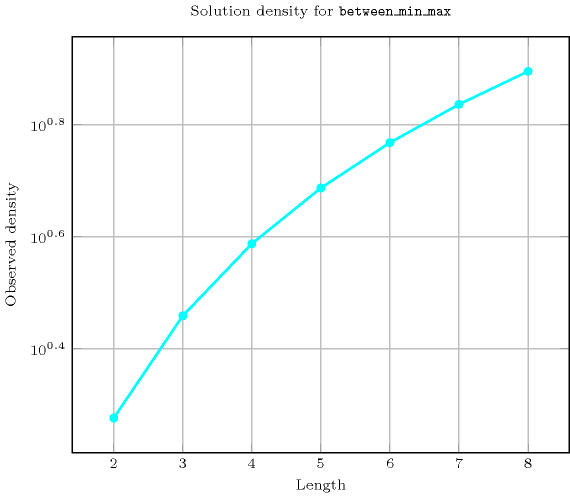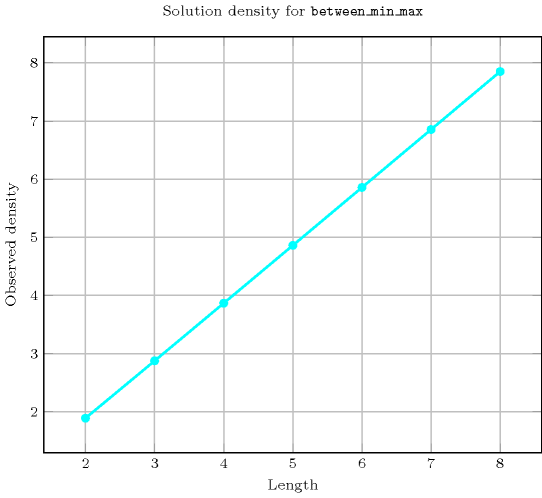Length ($n$)2345678
Total1718424173780668920114376608338051265
 Parameter value

0537369465170993127360926269505
17555436751102023181721537281919
25555937501113489201889941366849
3-375437501116191207858142649535
4--3696751113489207858142915649
5---4651102023201889942649535
6----70993181721541366849
7-----127360937281919
8------26269505

Solution count for $\mathrm{𝚋𝚎𝚝𝚠𝚎𝚎𝚗}_\mathrm{𝚖𝚒𝚗}_\mathrm{𝚖𝚊𝚡}$: domains $0..n$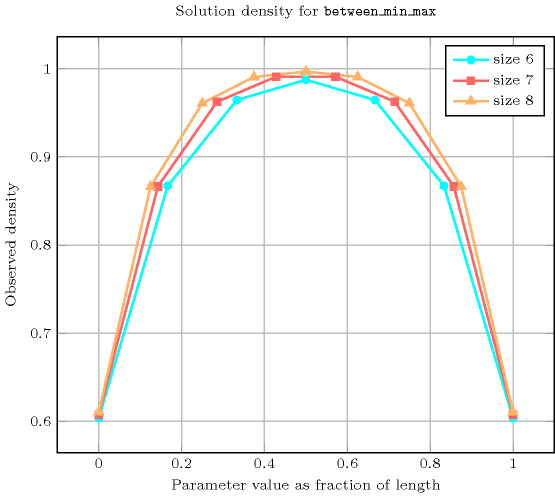Used in
Keywords
Derived Collection
$\mathrm{𝚌𝚘𝚕}\left(\mathrm{𝙸𝚃𝙴𝙼}-\mathrm{𝚌𝚘𝚕𝚕𝚎𝚌𝚝𝚒𝚘𝚗}\left(\mathrm{𝚟𝚊𝚛}-\mathrm{𝚍𝚟𝚊𝚛}\right),\left[\mathrm{𝚒𝚝𝚎𝚖}\left(\mathrm{𝚟𝚊𝚛}-\mathrm{𝚅𝙰𝚁}\right)\right]\right)$
Arc input(s)

$\mathrm{𝙸𝚃𝙴𝙼}$ $\mathrm{𝚅𝙰𝚁𝙸𝙰𝙱𝙻𝙴𝚂}$

Arc generator
$\mathrm{𝑃𝑅𝑂𝐷𝑈𝐶𝑇}$$↦\mathrm{𝚌𝚘𝚕𝚕𝚎𝚌𝚝𝚒𝚘𝚗}\left(\mathrm{𝚒𝚝𝚎𝚖},\mathrm{𝚟𝚊𝚛𝚒𝚊𝚋𝚕𝚎𝚜}\right)$

Arc arity
Arc constraint(s)
$\mathrm{𝚒𝚝𝚎𝚖}.\mathrm{𝚟𝚊𝚛}\ge \mathrm{𝚟𝚊𝚛𝚒𝚊𝚋𝚕𝚎𝚜}.\mathrm{𝚟𝚊𝚛}$
Graph property(ies)
$\mathrm{𝐍𝐀𝐑𝐂}$$\ge 1$

Graph class
 $•$$\mathrm{𝙰𝙲𝚈𝙲𝙻𝙸𝙲}$ $•$$\mathrm{𝙱𝙸𝙿𝙰𝚁𝚃𝙸𝚃𝙴}$ $•$$\mathrm{𝙽𝙾}_\mathrm{𝙻𝙾𝙾𝙿}$

Arc input(s)

$\mathrm{𝙸𝚃𝙴𝙼}$ $\mathrm{𝚅𝙰𝚁𝙸𝙰𝙱𝙻𝙴𝚂}$

Arc generator
$\mathrm{𝑃𝑅𝑂𝐷𝑈𝐶𝑇}$$↦\mathrm{𝚌𝚘𝚕𝚕𝚎𝚌𝚝𝚒𝚘𝚗}\left(\mathrm{𝚒𝚝𝚎𝚖},\mathrm{𝚟𝚊𝚛𝚒𝚊𝚋𝚕𝚎𝚜}\right)$

Arc arity
Arc constraint(s)
$\mathrm{𝚒𝚝𝚎𝚖}.\mathrm{𝚟𝚊𝚛}\le \mathrm{𝚟𝚊𝚛𝚒𝚊𝚋𝚕𝚎𝚜}.\mathrm{𝚟𝚊𝚛}$
Graph property(ies)
$\mathrm{𝐍𝐀𝐑𝐂}$$\ge 1$

Graph class
 $•$$\mathrm{𝙰𝙲𝚈𝙲𝙻𝙸𝙲}$ $•$$\mathrm{𝙱𝙸𝙿𝙰𝚁𝚃𝙸𝚃𝙴}$ $•$$\mathrm{𝙽𝙾}_\mathrm{𝙻𝙾𝙾𝙿}$

Graph model

Parts (A) and (B) of Figure 5.50.1 respectively show the initial and final graph associated with the second graph constraint of the first example of the Example slot. Since we use the $\mathrm{𝐍𝐀𝐑𝐂}$ graph property, the two arcs of the final graph are stressed in bold. The constraint holds since 3 is greater than 1 and since 3 is less than 8.

##### Figure 5.50.1. Initial and final graph of the $\mathrm{𝚋𝚎𝚝𝚠𝚎𝚎𝚗}_\mathrm{𝚖𝚒𝚗}_\mathrm{𝚖𝚊𝚡}$ constraint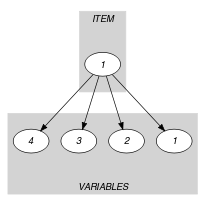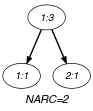(a) (b)
Automaton

Figure 5.50.2 depicts the automaton associated with the $\mathrm{𝚋𝚎𝚝𝚠𝚎𝚎𝚗}_\mathrm{𝚖𝚒𝚗}_\mathrm{𝚖𝚊𝚡}$ constraint. To each pair $\left(\mathrm{𝚅𝙰𝚁},{\mathrm{𝚅𝙰𝚁}}_{i}\right)$, where ${\mathrm{𝚅𝙰𝚁}}_{i}$ is a variable of the collection $\mathrm{𝚅𝙰𝚁𝙸𝙰𝙱𝙻𝙴𝚂}$ corresponds a signature variable ${S}_{i}$. The following signature constraint links $\mathrm{𝚅𝙰𝚁}$, ${\mathrm{𝚅𝙰𝚁}}_{i}$ and ${S}_{i}$: $\left(\mathrm{𝚅𝙰𝚁}<{\mathrm{𝚅𝙰𝚁}}_{i}⇔{S}_{i}=0\right)\wedge \left(\mathrm{𝚅𝙰𝚁}={\mathrm{𝚅𝙰𝚁}}_{i}⇔{S}_{i}=1\right)\wedge \left(\mathrm{𝚅𝙰𝚁}>{\mathrm{𝚅𝙰𝚁}}_{i}⇔{S}_{i}=2\right)$.

##### Figure 5.50.2. Automaton of the $\mathrm{𝚋𝚎𝚝𝚠𝚎𝚎𝚗}_\mathrm{𝚖𝚒𝚗}_\mathrm{𝚖𝚊𝚡}$ constraint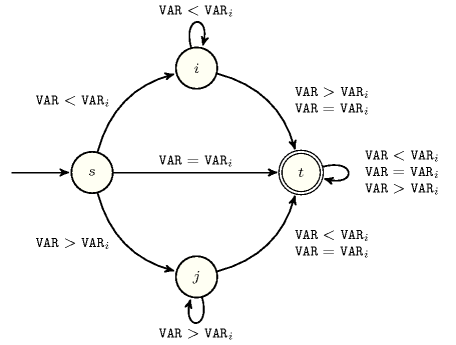##### Figure 5.50.3. Hypergraph of the reformulation corresponding to the automaton of the $\mathrm{𝚋𝚎𝚝𝚠𝚎𝚎𝚗}_\mathrm{𝚖𝚒𝚗}_\mathrm{𝚖𝚊𝚡}$ constraint